# Local Binary Pattern for Local Texture Feature Extraction

By Matthew Millar R&D Scientist at ユニファ

This blog will look at how to build a Local Binary Pattern feature extractor for computer vision tasks.

### Local Binary Pattern:

What is LBP
LBP is one of many feature extractors. HOG, SIFT, SURF, FAST, DoG, etc... are all similar but do slightly different things. What makes LBP different is, its main goal is to be used for a texture descriptor on a local level. This gives a local representation of any texture of an image. This is done by comparing a pixel with the surrounding pixels. For each pixel in an image, the surrounding x number of pixels will be looked at. X can be determined and adjusted as needed. The LBP value for every pixel is calculated to its neighbors. If the center pixel is greater than or equal it's neighbor's values, then it will be set to 1, else it will be set to 0.

From the above talbe, you can see how each cell gets calculated. From this point this 2D array will be flattened to a 1D array like this:

This will giveSo 71 will be in the output image. This process will be done for every single pixel in the image.

This talbe shows how each cell is calculated:

The basic idea behind this is to calculate each value of the 1D array at each index.
The value is determined by the position of the index in the array. If the value at the index is a 1, then value calculated to bewhere i is the index position. If the value at the index is 0, then the value is set to a 0 regardless of the index position. Then you sum the results of the whole 1D array to get the center pixel value.
To get the feature vectors from this, you have to calculate a histogram first.
This will be a histogram of 256 bins as the values of the LBP can range from 0 to 255.

### Python Implementation:

OpenCV does have an LBP available, but it is meant for facial recognition and would not be appropriate for getting textures off clothing or environments. The use of the sklearn’s model can be very useful then for this project.
Let see how to implement it.

from skimage import feature
from sklearn.svm import LinearSVC
from sklearn.linear_model import LogisticRegression
import numpy as np
import cv2
import os

class LBP:
# Constructor
# Needs the radius and number of points for the outer radius
self.numPoints = numPoints
# Compute the actual lbp
def calculate_histogram(self, image, eps=1e-7):
# Create a 2D array size of the input image
lbp = feature.local_binary_pattern(image, self.numPoints,
method="uniform")
# Make feature vector
#Counts the number of time lbp prototypes appear
(hist, _) = np.histogram(lbp.ravel(),
bins= np.arange(0, self.numPoints + 3),
range=(0, self.numPoints +2))
hist = hist.astype("float")
hist /= (hist.sum() + eps)

return hist

# Create the lbp
loc_bi_pattern = LBP(12,12)
x_train = []
y_train = []

image_path = "LBPImages/"
train_path = os.path.join(image_path, "train/")
test_path = os.path.join(image_path, "test/")

for folder in os.listdir(train_path):
folder_path = os.path.join(train_path, folder)
print(folder_path)
for file in os.listdir(folder_path):
image_file = os.path.join(folder_path, file)
image = cv2.resize(image,(300,300))
gray = cv2.cvtColor(image, cv2.COLOR_BGR2GRAY)
hist = loc_bi_pattern.calculate_histogram(gray)
# Add the data to the data list
x_train.append(hist)
y_train.append(folder)


After then you can choose whichever model you want to train on. SVM I think would be best, but logistic regression or Naive Bayes could work also. It would be fun to play around with a few options to see which works best.
I trained and tested my code on images of metal and wood textures.
The results are pretty good for something so simple:

Pretty straight forward seeing we are using sklearn's implementation. All we really need to do is create the histograms to get out the feature vectors for each image. This can allow for you to then classify other images that have similar textures on them.
As you can see it works pretty well. The first “wood” image is actually metal siding, but I wanted to see how well it does one something that is very difficult to determine. This misclassification could be due to the overall image looking similar to that of wood flooring texture and not of metal textures. Even a human might have the same issue with this using a black and white photo.

### Conclusion:

The ability to extract small scale or fine grain details makes LBP a very handy tool for computer vision tasks. But, one issue is that LBP cannot capture at different scales which causes it to miss out on a global scale features. This can be overcome by using different implementations of LBP which can handle different neighborhood sizes which allows for better control over the scale. Depending on your need the use of a fixed scale or changing one might change.

https://www.pexels.com/

# AndroidXに移行してみた

こんにちは、Androidエンジニアのあいばです。

じめじめと嫌な天気が続いていますが、いかがお過ごしでしょうか。

## AndroidXについて

AndroidX の概要  |  Android Developers

## 今回のゴール

AndroidX移行したプロジェクトをCircleCIでビルド＆配信できること

## 早速やってみる

### Auto migration

まずはこちらを参考にauto migrationを実行しました。

Migrating to AndroidX  |  Android Developers

Android Studioのメニューバーから [Refactor] > [Migrate to AndroidX] を実行するとgradle.propertiesに次の2つのフラグが追加され、Android Studio のビルドシステムにより依存関係も自動的に移行されます。

android.useAndroidX=true
android.enableJetifier=true


#### 追加で必要だった対応

• DataBindingについて次のエラーが発生
ERROR: Data Binding annotation processor version needs to match the Android Gradle Plugin version.
You can remove the kapt dependency androidx.databinding:databinding-compiler:1.0.0 and Android Gradle
Plugin will inject the right version.

次の行を削除する事で解決しました。

    kapt 'androidx.databinding:databinding-compiler:1.0.0'


Android Studio Canary8から不要になっていたそうです。
DataBindingのkaptを書かなくても良くなった - Kenji Abe - Medium

• 次のようにexcludeなどでサポートライブラリを指定している箇所は自動で移行されないため必要に応じて削除、修正が必要です。
    implementation("com.squareup.picasso:picasso:$picassoVersion") { exclude group: "com.android.support", module: "exifinterface" }  • 自動で移行されたライブラリはバージョン1.0.0と指定されているのでその時の最新バージョンに更新します。 ##### src • importは正しく修正されていますが、コード内でも完全修飾で参照してしまっているので修正します。 • RecyclerView.ItemDecoration.onDraw(Canvas, RecyclerView, RecyclerView.State)など引数がNonNullに変更されたoverrideメソッドでエラーになるので修正が必要です。 #### テスト ここまでの作業を終えてテストを実行したところ…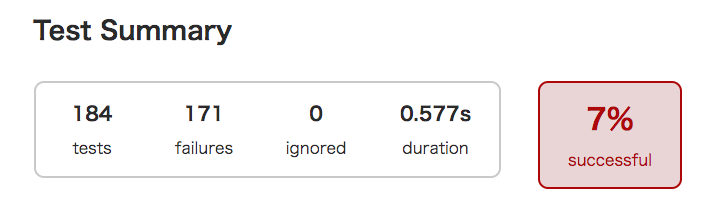見ての通り撃沈です。 Robolectricを利用しているテストが初期化で失敗していました。 Robolectricのバージョンが3.xと古かったため最新にして再びテスト実行します。 結果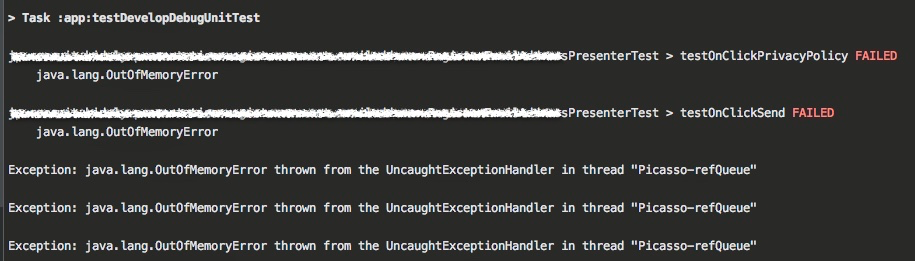今度は OutOfMemoryError となったので build.gradleに次の設定を追加します。 android { testOptions { unitTests.all { maxHeapSize = "1g" } } }  成功です！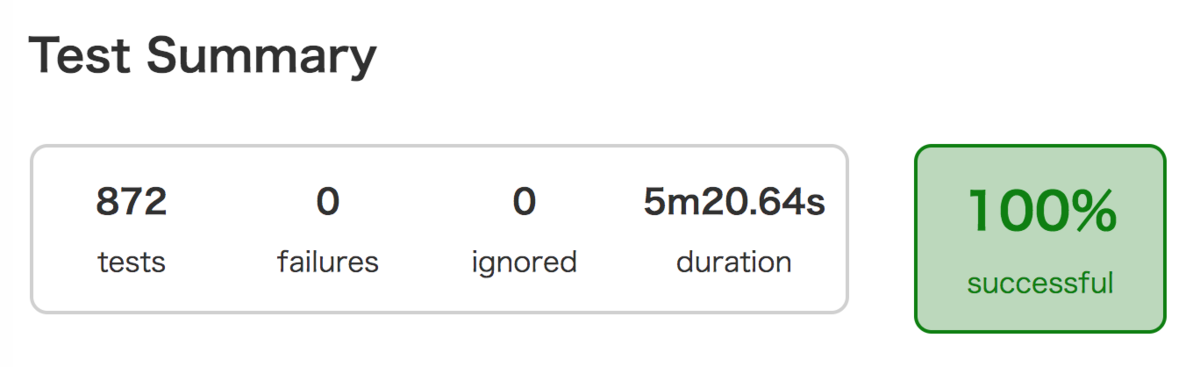#### CircleCIでビルド 満を持してCircleCIでビルドします！ 失敗です！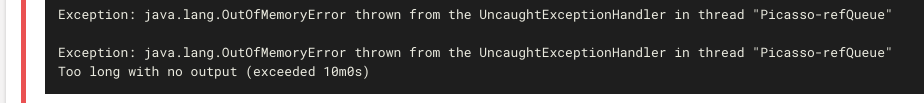テスト実行時に先ほど倒したはずのOutOfMemoryErrorが発生してます。 どうやらCircleCI 上のコンテナで使用できるメモリが不足しているようだったので、CircleCIのプランを見直しresource_classオプションを利用することにしました。 Configuring CircleCI - CircleCI 今度こそ成功です！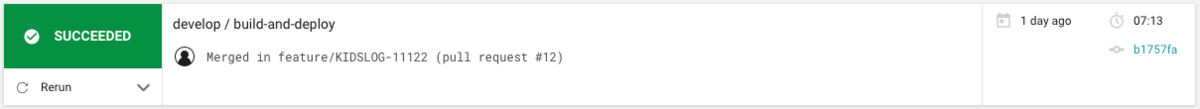# Hadoop from Start to Finish on Windows10 By Matthew Millar R&D Scientist at ユニファ This blog will be looking at how to set up and start a Hadoop server on windows as well as give some explanation as to what it is used for. ### What is Hadoop: Hadoop is a set of tools that can be used for easy processing and analyzing Big Data for a company and research. Hadoop gives you tools to manage, query, and share large amounts of data with people who are dispersed over a large geographical location. This means that teams in Tokyo can easily work with teams in New York, well not accounting for sleeping preferences. Hadoop gives a huge advantage over a traditional storage system, not only in the total amount of storage possible, but in flexibility, scalability, and speed of access to this data. ### Modules Hadoop is split up into 4 distinctive modules. Each module performs a certain task that is needed for the distributed system to function properly. A distributed system is a computer system that has its components separated over a network of different computers. This can be both data and processing power. The actions of the computers are coordinated by messages that are passed back and forth between each other. These systems are complex to set up and maintain but offer a very powerful network to process large amounts of data or run very expensive jobs quickly and efficiently. The first module is the Distributed Filesystem. The HDFS allows files to be stored, processed, shared and managed across a set of connected storage devices. HDFS is not like a regular operating file system and normally can be accessed by any supported OS which gives a great deal of freedom. The second module is MapReduce. There are two main functions that this module performs. Mapping is the act of reading in the data (or gathering it form each node). Mapping then puts all this data into a format that can be used for analysis. Reduce can be considered the place where all the logic is performed on the collected data. In other words, Mapping gets the data, Reducing analyzes it. Hadoop common is the third module. This module consists of a set of Java tools that each OS needs to access and read the data that is stored in the HDFS. The final module is YARN which is the system management that manages the storing of the data and running of task/analysis of the data. ### Little More Detail: What is a namenode? A namenode stores all the metadata of all the files in the HDFS. This includes permissions, names, and block locations. These blocks can be mapped to each datanode. The namenode is also responsible for managing the datanode, i.e. where it is saved, which blocks are on which node, etc… A datanode, aka a slave node, is the node that actually stores and retrieves blocks of information requested by the namenode. ### Installation: Now with the background out of the way lets try to install the system on Windows 10. Step 1: Download Hadoop Binaries from here Apache Download Mirrors Step 2: Make its own folder in the C drive to keep things tidy and make sure that it is easy to find. NOTE DO NOT PUT ANY SPACES AS IT CAN CAUSE SOME VARIABLES TO IMPROPERLY EXPAND.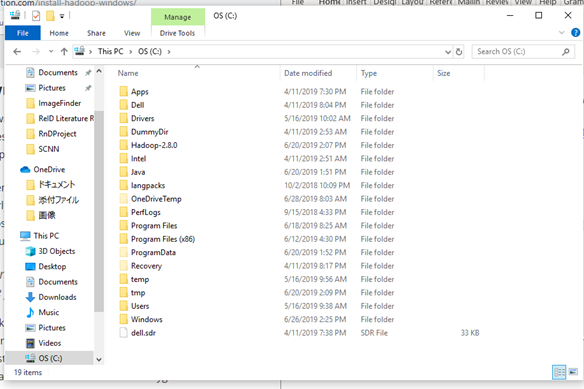Folder Setup Step 3: Unpack the tar.gz file (I suggest 7 zip as it works on windows and is free) Step 4: To run it on Windows, you need a windows compatible binary from this repo https://github.com/ParixitOdedara/Hadoop. You can just download the bin folder and copy all the files from the downloaded bin to Hadoop's bin (replace any files if needed). Simple right. Step 5: Create a folder called data and, in this folder, create two others called datanode and namenode. The datanode will hold all the data that is assigned to it. The namenode is the master node which holds the metadata for the datanode (i.e. which data node the 64mb blocks is located on)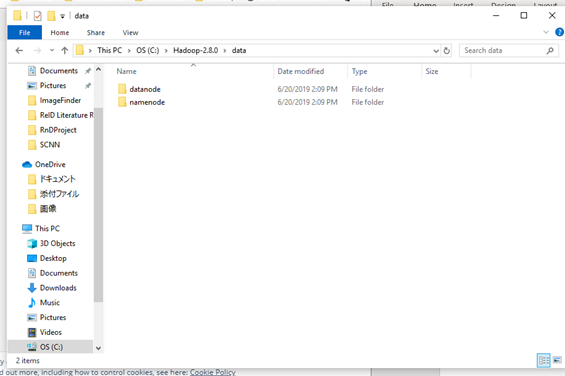Datanode Namenode Step 6: Set up Hadoop Environment variables like so: HADOOP_HOME=”C:\BigData\hadoop-2.9.1\bin” JAVA_HOME=<Root of your JDK installation>”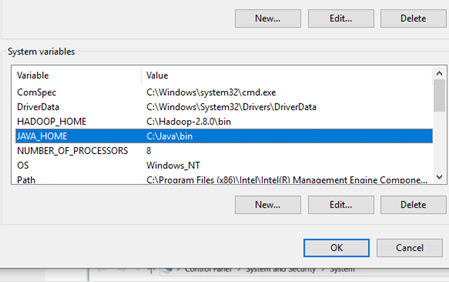Environment Vars And add it to your path variables like this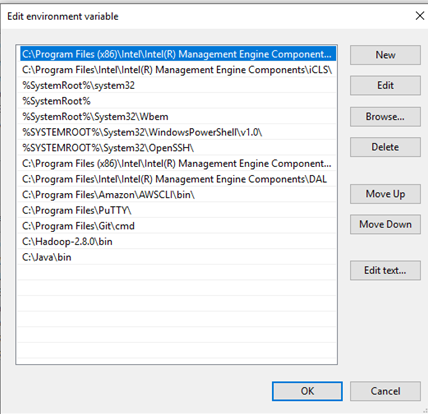Path Vars Step 7: Editing several configuration files. First up is the: Ect\hadoop\hadoop-env.cmd set HADOOP_PREFIX=%HADOOP_HOME% set HADOOP_CONF_DIR=%HADOOP_PREFIX%\etc\hadoop set YARN_CONF_DIR=%HADOOP_CONF_DIR% set PATH=%PATH%;%HADOOP_PREFIX%\bin  Next, let's look at: Ect\hadoop\core-site.xml <configuration> <property> <name>fs.default.name</name> <value>hdfs://0.0.0.0:19000</value> </property> </configuration>  Then the ect\hadoop\hdfs-site.xml <configuration> <property> <name>dfs.replication</name> <value>1</value> </property> <property> <name>dfs.namenode.name.dir</name> <value>C:\BigData\hadoop-2.9.1\data\namenode</value> </property> <property> <name>dfs.datanode.data.dir</name> <value>C:\BigData\hadoop-2.9.1\data\datanode</value> </property> </configuration>  And now the: Ect\hadoop\mapred-site.xml <configuration> <property> <name>mapreduce.job.user.name</name> <value>%USERNAME%</value> </property> <property> <name>mapreduce.framework.name</name> <value>yarn</value> </property> <property> <name>yarn.apps.stagingDir</name> <value>/user/%USERNAME%/staging</value> </property> <property> <name>mapreduce.jobtracker.address</name> <value>local</value> </property> </configuration>  ### Running Hadoop On the first time you start up you need to run I a cmd write: Hadoop namenode -format This set up your namenode and gets Hadoop running. Now cd into your sbin folder and type start-all.cmd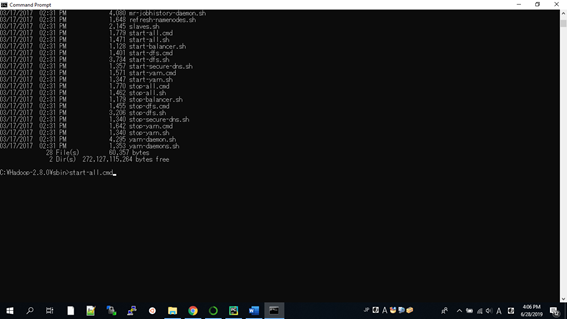sbin This will open up 4 other screens like this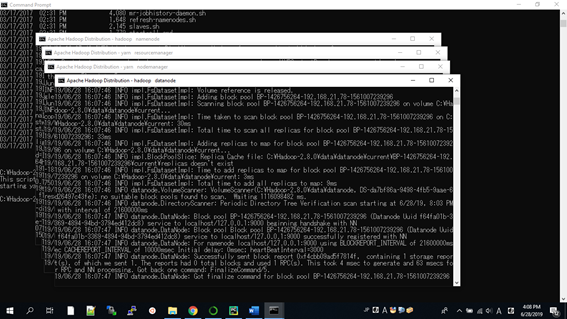Hadoop First run Their names are; namenode, datanode, nodemanager, and resourcemanager. And now we can look at Hadoop in a browser The resource manager is http://localhost:8088/cluster/cluster This is what you should be greeted with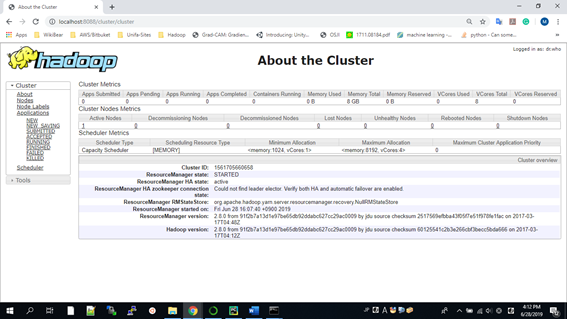Hadoop Homepage Here are the links for the; node manager, datanode, and a manager for Hadoop. http://localhost:8042/node http://localhost:50070/dfshealth.html#tab-overview http://localhost:50075/datanode.html ### Working with Hadoop Now we can finally start to work or using Hadoop after all this set and configuration. Open a cmd To insert data into the HDFS use the following fs -mkdir /user/input fs -put /home/file.txt /user/input fs -ls /user/input  To retrieve the Data from HDFS use the following fs -cat /user/output/outfile fs -get /user/output/ /home/hadoop_tp/  And finally shut down the system. stop-dfs.sh  And that’s it, we managed to install and use Hadoop. Now this is a very simple way of doing it and there may be better approaches like using Dockers, or commercial versions which are much easier to use and setup, but learning how to set it up and run it from scratch is a good experience. ### Conclusion We learned that it was very complex to set up and configure all of Hadoop. But with all the power it can bring to Big Data analysis as well as large data sets that are used for AI training and testing, Hadoop can be a very powerful tool in any researcher, data scientist, and business intelligence analyst. A potential use of Hadoop form image analysis where you have images that are stored in different sources or if you want to use a standard set of images, but the number of images is too large to store locally in a traditional storage solution. Using Hadoop, one can establish a feeding reducer that can then be used in a data generator method in a Keras model. The potentially one can have an endless stream of data from an extremely large dataset, thus giving almost unlimited data. This approach can also be used to get numerical data that is stored on a Hadoop system. Now you do not have to download the data directly just use Hadoop to query and do preprocessing of the data before you feed it into your model. This can save time and energy when working with distributed systems. # SIFT for Feature Extraction in a Image in Python ### Feature Extraction SIFT (Scale Invariant Feature Transform) Why am I making this post? Well, I basically needed to make my own SIFT algorithm as there is no free one in OpenCV anymore, well at least 3.0+. For computer vision, one of the most basic ideas is to extract information from an image. This is feature extraction. There are different levels of features mainly global and local features. This blog will look at SIFT which is a local feature extractor. This is done by finding key points or areas of great change then adds quantitative information or descriptors that can then be used in a more complex task like object detection. Ideally, these key points should be able to be uniquely identified in various images regardless of transformations or changes in the image. Why Python? Yes, it's not the best in speed for this, and after running the code it takes a hot minute for it to do the feature extraction. But, I can easily use it in any computer vision project that I have now and it plugs and play no problem. ### How does SIFT work? First, you give SIFT a picture to work with, we will be using an image I took of a dog from when I went dog sledding in Finland. Step1: Double the size of your image both using bilinear interpolation. Step 2: Blur the image using Gaussian Convolution. Step 3: Preform more convolutions using Standard Deviation. Step 4: Downsample each image. Step 5: Restart the convolution again. Continue this until the image is too small to perform these steps anymore. This is called a scale-space which will help simulate many different scales that an image can come in (i.e. from small to larger and everything in between). After the convolution, we will have to get the Laplacian for each scale space. This gives a grey scale value for each element in the image. The max values for the Laplacian will then be our key points. The max pixel will be a pixel whose value is larger than all its surrounding other pixels. This can be extended to several pixels or even a larger area depending on your needs. We can refine the key point results in the scale space by using a Quadratic Taylor expansion. Along with this, the identification of key points that lie on the edge of an object should also be removed as they are poor key points as they are not unique to translations of an image and are parallel to the edge direction. The point of keypoint extractions is not to find an edge of an object, but rather to find unique features of the image which may or may not lie on the edge of a target. That is the difference between edge detection and key point detection. Finally, SIFT will give a reference orientation to each key point. Then the gradient of the image will be calculated by finite differences. Then smooth the gradients of the image using box blurs. This will allow for the remaining points that exceed a certain value/threshold to be kept. Another key point will be discarded. After all of that, we have a list of final key points that we can create the descriptors for. This is done by creating a histogram of the gradient directions for each key point. This will not just make one histogram as it will make several around in a circle of the pixel where the histogram corresponds to the center pixel. Each gradient is a circle shape (rather than a box as used previously), and any key point that cannot create a full circle will be discarded. So, after all of this, we now are left with a set of key points that are local features that can help us identify unique objects in images. This can then be used on its own for simple computer vision tasks like object identification, image slicing, and binding, or even image similarity for search engines. So, SIFT can be very useful if you know how and why it works. ### Code for SIFT Here is the pseudo code for getting SIFT for key point detection. Sift Code Find_Key_Points(image): Gaussian Smoothing (image) Downsample the image Make Gaussians Pyramids Create Downsample Gaussians Pyramids For each octave: Start Extrema detection. for each image sample at each scale: find the gradient magnitude find the orientation Calculate each keypoints orientation Calculate each keypoints descriptor  As you can see the key points found in this image is not perfect, but the implementation of SIFT is not very easy. Also, this image has a lot of noise in it so it may look like the algorithm did not work, but it is working fine, but the changes are on such a small level that it is difficult to even see it.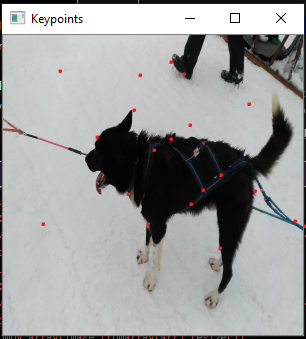DogSled Keypoints And for those who want to see here is the whole (all be it abridged) code import numpy as np from scipy import signal from scipy import misc from scipy import ndimage from scipy.stats import multivariate_normal from numpy.linalg import norm import numpy.linalg def sift_generate_keypoints(imagename, threshold): original = ndimage.imread(imagename, flatten=True) s = 3 k = 2 ** (1.0 / s) # Standard deviations for Gaussian smoothing vector_1 = np.array([1.3, 1.6, 1.6 * k, 1.6 * (k ** 2), 1.6 * (k ** 3), 1.6 * (k ** 4)]) vector_2 = np.array([1.6 * (k ** 2), 1.6 * (k ** 3), 1.6 * (k ** 4), 1.6 * (k ** 5), 1.6 * (k ** 6), 1.6 * (k ** 7)]) ... vector_total = np.array([1.6, 1.6 * k, 1.6 * (k ** 2), 1.6 * (k ** 3), 1.6 * (k ** 4), 1.6 * (k ** 5), 1.6 * (k ** 6), 1.6 * (k ** 7), 1.6 * (k ** 8), 1.6 * (k ** 9), 1.6 * (k ** 10), 1.6 * (k ** 11)]) # Downsampling images doubled = misc.imresize(original, 200, 'bilinear').astype(int) normal = misc.imresize(doubled, 50, 'bilinear').astype(int) halved = misc.imresize(normal, 50, 'bilinear').astype(int) quartered = misc.imresize(halved, 50, 'bilinear').astype(int) # Initialize Gaussian pyramids pyramid_l_1 = np.zeros((doubled.shape, doubled.shape, 6)) pyramid_l_2 = np.zeros((normal.shape, normal.shape, 6)) pyramid_l_3 = np.zeros((halved.shape, halved.shape, 6)) pyramid_l_4 = np.zeros((quartered.shape, quartered.shape, 6)) # Gaussian pyramids for i in range(0, 6): pyramid_l_1[:,:,i] = ndimage.filters.gaussian_filter(doubled, kvec1[i]) ... pyramid_l_4[:,:,i] = misc.imresize(ndimage.filters.gaussian_filter(doubled, kvec4[i]), 1.0 / 8.0, 'bilinear') # Difference-of-Gaussians (DoG) pyramids dog_pyrd_l_1 = np.zeros((doubled.shape, doubled.shape, 5)) ... dog_pyrd_l_4 = np.zeros((quartered.shape, quartered.shape, 5)) # Construct DoG pyramids for i in range(0, 5): dog_pyrd_l_1[:,:,i] = pyrlvl1[:,:,i+1] - pyrlvl1[:,:,i] ... dog_pyrd_l_4[:,:,i] = pyrlvl4[:,:,i+1] - pyrlvl4[:,:,i] # extrema locations pyramids extrem_loc_l_1 = np.zeros((doubled.shape, doubled.shape, 3)) ... extrem_loc_l_4 = np.zeros((quartered.shape, quartered.shape, 3)) for i in range(1, 4): for j in range(80, doubled.shape - 80): for k in range(80, doubled.shape - 80): if np.absolute(dog_pyrd_l_1[j, k, i]) < threshold: continue maxbool = (dog_pyrd_l_1[j, k, i] > 0) minbool = (dog_pyrd_l_1[j, k, i] < 0) for di in range(-1, 2): for dj in range(-1, 2): for dk in range(-1, 2): if di == 0 and dj == 0 and dk == 0: continue maxbool = maxbool and (dog_pyrd_l_1[j, k, i] > dog_pyrd_l_1[j + dj, k + dk, i + di]) minbool = minbool and (dog_pyrd_l_1[j, k, i] < dog_pyrd_l_1[j + dj, k + dk, i + di]) if not maxbool and not minbool: break if not maxbool and not minbool: break if not maxbool and not minbool: break if maxbool or minbool: dx = (dog_pyrd_l_1[j, k+1, i] - dog_pyrd_l_1[j, k-1, i]) * 0.5 / 255 dy = (dog_pyrd_l_1[j+1, k, i] - dog_pyrd_l_1[j-1, k, i]) * 0.5 / 255 ds = (dog_pyrd_l_1[j, k, i+1] - dog_pyrd_l_1[j, k, i-1]) * 0.5 / 255 dxx = (dog_pyrd_l_1[j, k+1, i] + dog_pyrd_l_1[j, k-1, i] - 2 * dog_pyrd_l_1[j, k, i]) * 1.0 / 255 dyy = (dog_pyrd_l_1[j+1, k, i] + dog_pyrd_l_1[j-1, k, i] - 2 * dog_pyrd_l_1[j, k, i]) * 1.0 / 255 dss = (dog_pyrd_l_1[j, k, i+1] + dog_pyrd_l_1[j, k, i-1] - 2 * dog_pyrd_l_1[j, k, i]) * 1.0 / 255 dxy = (dog_pyrd_l_1[j+1, k+1, i] - dog_pyrd_l_1[j+1, k-1, i] - dog_pyrd_l_1[j-1, k+1, i] + dog_pyrd_l_1[j-1, k-1, i]) * 0.25 / 255 dxs = (dog_pyrd_l_1[j, k+1, i+1] - dog_pyrd_l_1[j, k-1, i+1] - dog_pyrd_l_1[j, k+1, i-1] + dog_pyrd_l_1[j, k-1, i-1]) * 0.25 / 255 dys = (dog_pyrd_l_1[j+1, k, i+1] - dog_pyrd_l_1[j-1, k, i+1] - dog_pyrd_l_1[j+1, k, i-1] + dog_pyrd_l_1[j-1, k, i-1]) * 0.25 / 255 dD = np.matrix([[dx], [dy], [ds]]) H = np.matrix([[dxx, dxy, dxs], [dxy, dyy, dys], [dxs, dys, dss]]) x_hat = numpy.linalg.lstsq(H, dD) D_x_hat = dog_pyrd_l_1[j, k, i] + 0.5 * np.dot(dD.transpose(), x_hat) r = 10.0 if ((((dxx + dyy) ** 2) * r) < (dxx * dyy - (dxy ** 2)) * (((r + 1) ** 2))) and (np.absolute(x_hat) < 0.5) and (np.absolute(x_hat) < 0.5) and (np.absolute(x_hat) < 0.5) and (np.absolute(D_x_hat) > 0.03): extrem_loc_l_1[j, k, i - 1] = 1 #........ # Repeat for each octave # Gradient magnitude and orientation grad_mag_ori_l_1 = np.zeros((doubled.shape, doubled.shape, 3)) ... grad_mag_ori_l_4 = np.zeros((quartered.shape, quartered.shape, 3)) ori_pyramid_l_1 = np.zeros((doubled.shape, doubled.shape, 3)) ... ori_pyramid_l_4 = np.zeros((quartered.shape, quartered.shape, 3)) for i in range(0, 3): for j in range(1, doubled.shape - 1): for k in range(1, doubled.shape - 1): grad_mag_ori_l_1[j, k, i] = ( ((doubled[j+1, k] - doubled[j-1, k]) ** 2) + ((doubled[j, k+1] - doubled[j, k-1]) ** 2) ) ** 0.5 ori_pyramid_l_1[j, k, i] = (36 / (2 * np.pi)) * (np.pi + np.arctan2((doubled[j, k+1] - doubled[j, k-1]), (doubled[j+1, k] - doubled[j-1, k]))) # Repeat for each orientation pyramid extr_sum = int(np.sum(extrem_loc_l_1) + np.sum(extrem_loc_l_2) + np.sum(extrem_loc_l_3) + np.sum(extrem_loc_l_4)) keypoints = np.zeros((extr_sum, 4)) #Key point calculation count = 0 for i in range(0, 3): for j in range(80, doubled.shape - 80): for k in range(80, doubled.shape - 80): if extrem_loc_l_1[j, k, i] == 1: gaussian_window = multivariate_normal(mean=[j, k], cov=((1.5 * vector_total[i]) ** 2)) two_sd = np.floor(2 * 1.5 * vector_total[i]) orient_hist = np.zeros([36,1]) for x in range(int(-1 * two_sd * 2), int(two_sd * 2) + 1): ylim = int((((two_sd * 2) ** 2) - (np.absolute(x) ** 2)) ** 0.5) for y in range(-1 * ylim, ylim + 1): if j + x < 0 or j + x > doubled.shape - 1 or k + y < 0 or k + y > doubled.shape - 1: continue weight = grad_mag_ori_l_1[j + x, k + y, i] * gaussian_window.pdf([j + x, k + y]) bin_idx = np.clip(np.floor(ori_pyramid_l_1[j + x, k + y, i]), 0, 35) orient_hist[int(np.floor(bin_idx))] += weight maxval = np.amax(orient_hist) maxidx = np.argmax(orient_hist) keypoints[count, :] = np.array([int(j * 0.5), int(k * 0.5), vector_total[i], maxidx]) count += 1 orient_hist[maxidx] = 0 newmaxval = np.amax(orient_hist) while newmaxval > 0.8 * maxval: newmaxidx = np.argmax(orient_hist) np.append(keypoints, np.array([[int(j * 0.5), int(k * 0.5), vector_total[i], newmaxidx]]), axis=0) orient_hist[newmaxidx] = 0 newmaxval = np.amax(orient_hist) # Repeat for each octave # Create descriptors magnit_py = np.zeros((normal.shape, normal.shape, 12)) orient_py = np.zeros((normal.shape, normal.shape, 12)) for i in range(0, 3): magmax = np.amax(grad_mag_ori_l_1[:, :, i]) magnit_py[:, :, i] = misc.imresize(grad_mag_ori_l_1[:, :, i], (normal.shape, normal.shape), "bilinear").astype(float) magnit_py[:, :, i] = (magmax / np.amax(magnit_py[:, :, i])) * magnit_py[:, :, i] orient_py[:, :, i] = misc.imresize(ori_pyramid_l_1[:, :, i], (normal.shape, normal.shape), "bilinear").astype(int) orient_py[:, :, i] = ((36.0 / np.amax(orient_py[:, :, i])) * orient_py[:, :, i]).astype(int) for i in range(0, 3): magnit_py[:, :, i+3] = (magpyrlvl2[:, :, i]).astype(float) orient_py[:, :, i+3] = (oripyrlvl2[:, :, i]).astype(int) for i in range(0, 3): magnit_py[:, :, i+6] = misc.imresize(magpyrlvl3[:, :, i], (normal.shape, normal.shape), "bilinear").astype(int) orient_py[:, :, i+6] = misc.imresize(oripyrlvl3[:, :, i], (normal.shape, normal.shape), "bilinear").astype(int) for i in range(0, 3): magnit_py[:, :, i+9] = misc.imresize(grad_mag_ori_l_4[:, :, i], (normal.shape, normal.shape), "bilinear").astype(int) orient_py[:, :, i+9] = misc.imresize(ori_pyramid_l_4[:, :, i], (normal.shape, normal.shape), "bilinear").astype(int) descriptors = np.zeros([keypoints.shape, 128]) for i in range(0, keypoints.shape): for x in range(-8, 8): for y in range(-8, 8): theta = 10 * keypoints[i,3] * np.pi / 180.0 xrot = np.round((np.cos(theta) * x) - (np.sin(theta) * y)) yrot = np.round((np.sin(theta) * x) + (np.cos(theta) * y)) scale_idx = np.argwhere(vector_total == keypoints[i,2]) x0 = keypoints[i,0] y0 = keypoints[i,1] gaussian_window = multivariate_normal(mean=[x0,y0], cov=8) weight = magnit_py[int(x0 + xrot), int(y0 + yrot), scale_idx] * gaussian_window.pdf([x0 + xrot, y0 + yrot]) angle = orient_py[int(x0 + xrot), int(y0 + yrot), scale_idx] - keypoints[i,3] if angle < 0: angle = 36 + angle bin_idx = np.clip(np.floor((8.0 / 36) * angle), 0, 7).astype(int) descriptors[i, 32 * int((x + 8)/4) + 8 * int((y + 8)/4) + bin_idx] += weight descriptors[i, :] = descriptors[i, :] / norm(descriptors[i, :]) descriptors[i, :] = np.clip(descriptors[i, :], 0, 0.2) descriptors[i, :] = descriptors[i, :] / norm(descriptors[i, :]) return [keypoints, descriptors]  Now for a more real-world example. Getting data from the Market1501 data set that holds several images of people for different tasks. By running one of the images through the key point extractor, it allows for you to find the local features that are unique to the image itself.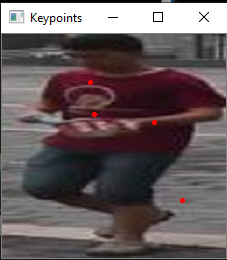PersonKeypointFrom the above picture you can see that a few of the key points are generated that look great, and one that is over on the street which is what you do not want. This is not the SIFT extractors fault it is looking over the whole image and not just the person. If you want a better result for a person's individual key points without the background mess, I would suggest using segmentation to create a mask and then use the results in the SIFT extractor. That way it will limit what is being looked at and will give better local features for each person. ### Conclusion This implementation is not the state of the art method for getting key points and is very susceptible to blurry or poor image quality, as well as background noise and noise in the image that you cannot detect yourself. This took a long while to implement and the results are not that great compared to the time it took to create this. In my opinion, I would look into other means of extracting local features like the segmentation of the image. ### Reference Otero, I. R., & Delbracio, M. (2014). Anatomy of the SIFT Method. Image Processing On Line,4, 370-396. doi:10.5201/ipol.2014.82 Market data set retrived from here: https://jingdongwang2017.github.io/Projects/ReID/Datasets/Market-1501.html # ユニファのエンジニア採用に興味がある人をベイズ推定で見極める #### はじめに こんにちわ、研究開発部の島田です。 先日、2019年度 人工知能学会全国大会が行われ、なんと弊社はプラチナスポンサーとして協賛し、ブースも出展させていただきました。当日は研究開発部のメンバーが総出で対応を行ったのですが、その際に色々な方から応援メッセージをいただけたので大変嬉しかったです。 学会の参加報告については、Podcastでも公開されていますのでご興味ある方はぜひこちらもどうぞ。 さて、会社としての学会参加の目的はいくつかあるわけですが、その中の一つとして”採用”があります。ですので、ブース対応するユニファ社員の関心事の一つとして、この人は「採用に興味があるのか、無いのか」になります。 採用に興味がある人であれば、出来るだけ短時間でかつ詳細に採用情報について知りたいはずです。そういう人に対しては的確な採用情報をなるべく早く与えてあげるべきですよね。 一方で、採用に興味が無い人に対して、採用情報ばかり提供してもお互いにとって良い時間の使い方にはならないはずです。 採用経験豊富な方であれば、目の前の人がどちらのタイプかをすぐに見極めるられるのでしょう。しかし、私みたいな初心者には無理です。。。それならエンジニアらしく、この直感的な判断を数値化してみよう！と思い立ちました。 ということで、前置きが長くなりましたが今回の記事は、学会のブースに来た人が採用に興味があるか否かを数値化してみたいと思います。 ※ただし、本記事で計算に使う各パラメータは全て架空のパラメータとします。 #### ベイズ推定 さて、目的の数値化のために、今回はベイズ推定を使いたいと思います。 ベイズ推定は、一般的には下記のように説明されます。 ベイズ確率の考え方に基づき、観測事象（観測された事実）から、推定したい事柄（それの起因である原因事象）を、確率的な意味で推論することを指す。 出典：Wikipedia 小難しく聞こえますが要するに、観測されたある事実を使って推定したい事柄を確率的に求める手法のことです。 ベイズ統計学という分野は昔からありましたが、最近また注目を浴びてきました。 例えば、MicrosoftやGoogleなどでもベイズ統計の技術を活かしていることで知られています。 ベイズ統計の特徴（強み）は以下があげられます。 • 事前知識を取り入れることで、少ないデータでもある程度の精度で推測可能。 • 入力情報によって瞬時にそして自動的に推測を更新する • 逐次合理性が成り立つ #### ベイズ推定の流れ はじめに今回のベイズ推定の処理の流れについて見ていきます。 大まかにはこういう流れで進めていきます。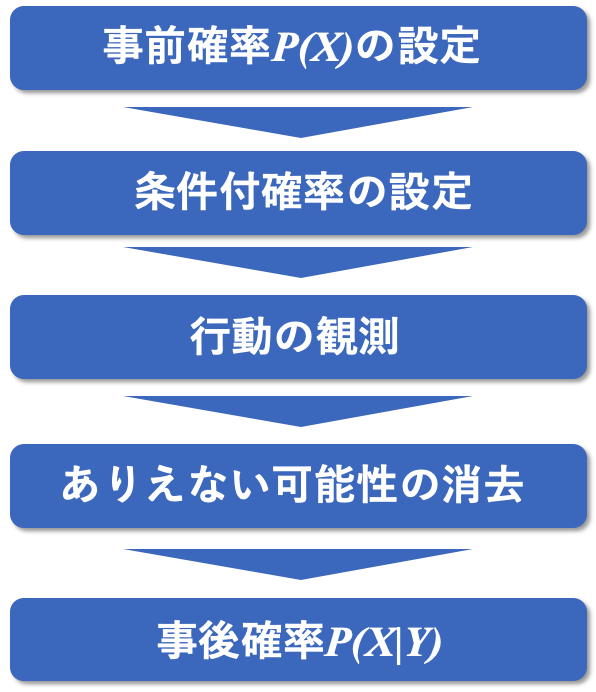#### 事前確率の設定 今回推定したいのは、ブースに来た人が採用に「興味ある」か「興味ない」かということです。そのために、推定の最初にすべきことはこの二つのタイプに対して、その割合を割り振ることです。この割合は経験に基づく設定の仕方と、そうでない割り当て方がありますが、ここでは、経験から数値が分かっているものとします。 例えば、経験的にブースを見に来た人の中で採用に「興味ある()」割合が10%(0.1)で、「興味ない()」割合が90%(0.9)だったとします。そうすると、事前確率は以下のように表せます。#### 条件付き確率の設定 次に、採用に興味あるかないかの二つのタイプに属する人の中で、それぞれどのくらいの割合でブースにいた弊社社員に「声をかけた」かを設定します。この条件付き確率では何らかの経験による裏付けや実験的な結果（数値）が必須です。ここでは、この結果（確率）も分かっているものとします。 例えば、「採用に興味がある人」は以下のような条件付き確率を設定します。 • 「採用に興味がある かつ 声をかける()」割合が80%(0.8) • 「採用に興味がある かつ 声をかけない()」割合が20%(0.2) 一方、「採用に興味がない人」は以下のように設定します。 • 「採用に興味がない かつ 声をかける()」割合が40%(0.4) • 「採用に興味がない かつ 声をかけない()」割合が60%(0.6) #### 行動の観測、ありえない可能性の消去 前項までの設定をし、実際に学会のブース対応したと仮定します。そしてありがたいことに、ある一人の方に声をかけられたとします。 これは一人の行動を実際に観測したことになり、「声かけしない」という可能性が消えて、確率が変化しました。 つまり、この実際の観測により、世界は以下の二つに限定されました。 1. 「採用に興味ある＆声かける」P(A and 声かけ) 2. 「採用に興味ない＆声かける」P(B and 声かけ) それぞれの確率を計算します。#### 事後確率を求める では、いよいよ本題である「声かけをしてきた人で採用に興味がある確率」を求めます。 前項にて、観測された事実からありえない可能性を消去し世界が変化したことで、確率の和が1にならなくなりました。 そこで、それぞれの確率の組を正規化（足して1になるようにする処理のこと）を行い、事後確率を求めます。この結果から、声をかけていただいた人が「採用に興味がある」確率は、約18%であると推定できました。 更に一つ大事なこととして、事前確率の設定の段階で「採用に興味がある人」と仮定したのは、10%でした。 ですが、「声をかける」という実際の事象を観測した後には、その人が採用に興味がある確率は、0.1から0.18と2倍弱にまで値が更新されました（これをベイズ更新と言います）。 このようにデータが与えられる度に値が更新されていくのもベイズ推定の一つの特徴でもあります。 #### おわりに ベイズ推定を簡単に応用したケースを紹介してみましたが、いかがでしたでしょうか。 ベイズ推定は実際のビジネスシーンにおいてもかなり幅広く応用できる手法ですので、今後も色々と試してみたいと思います。 また、冒頭でもお伝えした通り、今回のブース出展では大変多くの方に応援のメッセージを頂きました！皆さんの期待を裏切らないためにも日々精進していきたいと思います。 # iOSで光学的文字認識(OCR)を試してみる こんにちは、iOSエンジニアの大江です。 最近は梅雨入りしたと言っているのに全く雨が降らず、 安心しきっています。 安心したところにいきなりくるときがあるので、みなさん油断せずいきましょう！ ということで、雨に負けないよう楽しくOCRのお話をしていきたいと思います。 ### 前提 今回はいかにお金をかけずに手軽にやるかに重点を置いてみます！ ### iOSでのOCR iOSでOCRを行うには以下のような方法があります。 ライブラリ クラウド Google Cloud Vision API 端末 Tesseract OCR iOS SwiftOCR SwiftyTesseract Firebase MLKit(β) #### クラウド Google Cloud Vision API 言わずと知れた Google さんの出しているAPIです。 一度画像をアップロードする必要があるものの、 様々なファイルタイプに対応しており、言語も自動で判別してくれます。 1ヶ月に1000ユニットまでは無料ですが、それを越えると1000ユニットごとにお金がかかります。 #### 端末 Tesseract OCR iOS, SwiftOCR, SwiftyTesseract それぞれオープンソースのライブラリであり、 オフラインで利用することができます！ Tesseract OCR iOS はOCRエンジンにGoogle開発のTesseractを採用しており、情報量も多くあります。 しかし、学習データのバージョンが少し古いので精度に不安が残ります。 SwiftOCR はTesseractよりパフォーマンスが良いことをアピールしています。 ただし、短い英数字を読み取るのが得意らしいので、文章などを読み込むためにはTesseractの方が良いと説明しています。 また、日本語を読み取るためには学習データを作成する必要があります。 SwiftyTesseract は Tesseract OCR iOSと同じTesseract をOCRエンジンとして採用しています。 Tesseract OCR iOS との違いとしては、最新の安定バージョンのTesseractを使用しているので、 パフォーマンスが向上しています。 ただし、Tesseract本体のAPIに完全対応しているわけではないので、利用できない機能があります。 #### 両方 Firebase MLKit(β) Firebase MLKit(β) はβ版ながらクラウド版と端末版両方が用意されています。 しかし、端末版ではいくつかの言語しか対応していません。 日本語はというと・・・・・・・対応していません！！ なんてこった！！ 今回は日本語が試したいので、お休みしておいてもらいます。 ### 準備 今回はSwiftyTesseractを試してみたいと思います。 • macOS 10.14.5 • Xcode 10.2.1 （Swift 4.2） • CocoaPods 1.6.2 ### 実装 何はともあれ初めはこれです。 Xcodeプロジェクト作成しCocoaPodsをinitしてください。 やり方については、先達の方々の記事が検索すれば出てくると思うので、 そちらを参考にしていただければと思います。 #### SwiftyTesseractの導入 プロジェクトのPodfile を開いて以下の一行を追加します。 >pod 'SwiftyTesseract', '~> 2.0' 次にPodfileを保存して、下記のコマンドでインストールします。 $ pod install

これでSwiftyTesseractの導入は完了です！ 簡単ですね！

#### 学習データの追加

SwiftyTesseract ではTesseract用に用意されているデータを利用することができます。
Tesseract用の学習データには、次の3つのタイプが用意されています。

• tessdata_best (LSTM、正確性重視)
• tessdata_fast (LSTM、速度重視)
• tessdata (パターン認識とLSTMの組み合わせ)

SwiftyTesseractによると多くの場合tessdata_fast が最良になるとのことだったので、

1. tessdata_fastのリポジトリにアクセスし、jpn.traineddataダウンロードします。
2. Xcodeプロジェクトにtessdataフォルダを作成ます。
3. 作成したフォルダに、ダウンロードしてきたファイルを追加します。

これで日本語の学習データを利用する準備はできました。

この記事の一番初めの部分ですね！

#### OCR機能の実装

あとは実際にコードを書くだけです！
ViewController.swift で以下のように書いてあげてください。

import UIKit

// SwiftyTesseract のインポート
import SwiftyTesseract

class ViewController: UIViewController {

// swiftyTesseract インスタンスを生成
// この際引数で、言語の指定を行う。
let swiftyTesseract = SwiftyTesseract(language: RecognitionLanguage.japanese)

let filename = "sample.png"
guard let image = UIImage(named: filename) else { return }

swiftyTesseract.performOCR(on: image, completionHandler: { recognizedString in
guard let text = recognizedString else { return }
print(text)
})
}
}


#### 結果

まずまずの結果となっているのではないでしょうか。

っss 10Sで光学的文字認識(OcR)を試してみる
28
ロiOS     ロロSwift
こんにちは、iOSエンジニアの大江です。

たところにいきなりくるときがあるので、みなさん油断せずいきましょう!
ということで、雨に負けないよう楽しくOCRのお話をしていきたいと思います。

たところにいきなりくるときがあるので、みなさん油断せずいきましょう!
ということで、雨に負けないよう楽しくOCRのお話をしていきたいと思います。

i0SでのOCR
i0SでOCRを行うには以下のような方法があります。
ライブラリ

SwiftOCR
SwiftyTesseract
Firebase MLKitB)
クラウド

#### まとめ

また、記事には載せていませんが、背景色によって読み取り精度ががグッと下がったので、
そこも課題かなと思いました。

しかし、個人で試して楽しむだけなら手軽にできるので、
ぜひ試してみてください！

# Reinforcement Learning - Q-tables

### Q-Learning.

By Matthew Millar R&D Scientist at ユニファ

Q -learning is a model-free approach to reinforcement learning. This means that Q-learning does not rely on a model to make decisions based on an input, but instead uses a policy that shows the agent what actions to perform given a certain circumstance. Q-learning should find the best policy which gives an optimal solution to any finite Markov decision process. The FMDP can be described as:

Where X is the state space, A is the action space, and r is the reward function. The optimal solution is obtained by maximizing the total reward for any action and any change in state, starting with the current state. The best or optimal solution can be found for any finite problem given an unlimited exploration time combined with an exploration adaption to a policy. This optimal Q-function can be calculated as such:

The full equation and explanation can be found in Melo’s Convergence of Q-Learning: A Simple Proof :
Influences on how the agent will learn using Q-learning comes down to three real factors. Learning rate controls how much, or the rate in which new information replaces older information. If the learning rate is set to 0, then the agent will never learn anything new and will rely on previous knowledge to choose its actions. If the rate is set to 1, then the agent will only look at the most recent data and completely discard previous data.

The Discount factor is another variable that controls learning. The discount factor determines the weight of future rewards and how important or not these rewards will be. If it is set to 0, then the model will only consider instant rewards or the current reward and not look towards potential rewards of future actions. If the discount factor is set to 1, then the agent will work towards achieving the long-term highest reward.

The third and final variable used for learning the control is the initial conditions or Q0. Q-learning is an iterative program, it must have a starting condition before any changes take place to any state or environment. To help an agent explore options better, high initial values should be used regardless of which action is used. The update rules can control the values compared to other alternatives which can increase its total probability of being chosen. This will allow for each option to be fully explored and to aid in finding an optimal solution to each problem.

### Q-Tables:

Q-Learning runs off a table or matrix of state and actions. This Q-table starts out all values as 0 and after each episode, these values are updated accordingly. The matrix should take the size of both the state as well as the action size with a very basic form of this is a simple array [state, action]. The simplest method for creating a Q-table in python is this:

import numpy as np
# Initial Q-table
Q_table = np.zeros((state_size, action_size))


This Q-table will store every action and state that the agent will be in. Exploitation is done when an action that has been performed before and in a certain state is chosen which results in a reward (most likely the max possible future reward). Exploration is choosing a random action at any point in time and in any state. A basic method for this could be defined as below:

import random
epli = 0.4
def choose_action (epli)::
ran_float = random.random() # Generates a random float between [0,1]
if ran_float < epli:
#preform a random action
else:
get_action_from_qtable(current_state, q_table)


After choosing an action to perform and receiving a reward, the Q-table must be updated. The updating must be done after each action is performed. The updating phase will end at the conclusion of each episode. The basic flow of the update function will be:

Step 1: Get the current state of the agent.
Step 2: The agent takes action. (Picks random action or Pick action from Q-table)
Step 3: Update q-value.
An update rule for Q-values can be described as:

Q[state, action] = Q[state, action] + learning_rate * (reward + gamma * np.max(Q[new_state, :])) – Q[state, action]


This is a basic approach to adjust and update q-values in a Q-table.

Now for a simple example:
First lest look at the agent in the game:

class Agent:
def __init__(self, learning_rate = 0.1,
discount = 0.95,
exploration_rate = 1.0,
iterations = 1000):
# Q talbe for holding rewards
self.q_table = [[0,0,0,0,0], [0,0,0,0,0]]
self.learning_rate = learning_rate
self.discount = discount # Future rewards value to current rewards
self.exploration_rate = exploration_rate # Exploration rate
# This controls sift from exploration to exploation
self.exploration_delta = 1.0 / iterations

# Chose between explotation or exploration
def get_next_action(self, state):
if random.random() > self.exploration_rate:
return self.greedy_action(state)
else:
return self.random_action()

def greedy_action(self, state):
# Check to see if forward is best reward
if self.q_table[FORWARD][state] > self.q_table[BACKWARD][state]:
return FORWARD
elif self.q_table[BACKWARD][state] > self.q_table[FORWARD][state]:
return BACKWARD
# Rewards are equal, take random action
return FORWARD if random.random() < 0.5 else BACKWARD

def random_action(self):
return FORWARD if random.random() < 0.5 else BACKWARD

def update(self, old_state, new_state, action, reward):
old_value = self.q_table[action][old_state]
# best next action
future_action = self.greedy_action(new_state)
# reward for the best next action
future_reward = self.q_table[future_action][new_state]

# Main Q-table updating algorithm
new_value = old_value + self.learning_rate * (reward + self.discount * future_reward - old_value)
self.q_table[action][old_state] = new_value

# Finally shift our exploration_rate toward zero (less gambling)
if self.exploration_rate > 0:
self.exploration_rate -= self.exploration_delta


Then lets built the environment that he can walk through.

# Build the Environment
class EnvironmentSimulator:
def __init__(self, length=5, small=2, large=10):
self.length = length # Length of the environment
self.small = small  # reward for going back to the start
self.large = large  # reward for reaching the end
self.state = 0  # environment entry point

def take_action(self, action):
if action == BACKWARD:
reward = self.small
self.state = 0
elif action == FORWARD:
if self.state < self.length - 1:
self.state += 1
reward = 0
else:
reward = self.large
return self.state, reward
# Reset the environment
def reset(self):
self.state = 0
return self.state


This is a very simple array of 5 spaces. So basically, the agent can walk back a forth and get a reward for either reaching one end or the other.
Next, we will set up the environment and the main loop for running the simulation.

# Set up environment
environment = EnvironmentSimulator()
environment.reset()
# Scores
total_reward = 0
last_total = 0

for step in range(iterations):
# Store the current state in old state
old_state = environment.state
action = agent.get_next_action(old_state)
new_state, reward = environment.take_action(action)
agent.update(old_state, new_state, action, reward)

total_reward += reward
if step % 250 ==0:
performance = (total_reward - last_total) / 250.0
print("Step:{} Performance:{} Total-reward:{}".format(step, performance, total_reward))


### RI Hello World Revisited

Let's take a look back at the Cart Pole example from the previous blog,
tech.unifa-e.com
We did that with a Keras model, now we will do it with a q-table approach.

First we need to set up the Q-learning class

class QLearn:
def __init__(self, actions, epsilon, alpha, gamma):
self.q = {}
self.epsilon = epsilon
self.alpha = alpha
self.gamma = gamma
self.actions = actions


Next, we need a few methods for choosing actions and updating the tables:

    # Q learning function
def learnQ(self, state, action, reward, value):
old_value = self.q.get((state, action), None)
# If there are no values in the table add the reward
if old_value is None:
self.q[(state, action)] = reward
else:
self.q[(state, action)] = old_value + self.alpha * (value - old_value)

def chooseAction(self, state):
q = [self.getQ(state, a) for a in self.actions]
maxQ = max(q)

if random.random() < self.epsilon:
minQ = min(q); mag = max(abs(minQ), abs(maxQ))
q = [q[i] + random.random() * mag - .5 * mag for i in range(len(self.actions))]
maxQ = max(q)

count = q.count(maxQ)
if count > 1:
best = [i for i in range(len(self.actions)) if q[i] == maxQ]
i = random.choice(best)
else:
i = q.index(maxQ)
action = self.actions[i]
return action, q

def learn(self, s1, action, reward, s2):
maxqnew = max([self.getQ(s2, a) for a in self.actions])
self.learnQ(s1, action, reward, reward + self.gamma*maxqnew)


And now let's define the main method:

if __name__ == '__main__':
# Load the cart pole game from gym ai
env = gym.make('CartPole-v0')
# Vriables needed
max_number_of_steps = 200
last_time_steps = numpy.ndarray(0)
n_bins = 8
n_bins_angle = 10
max_number_episodes = 200
episode_number = 0
render = False

number_of_features = env.observation_space.shape
last_time_steps = numpy.ndarray(0)

# Simplfy the number of states as it is too large
cart_position = pandas.cut([-2.4, 2.4], bins=n_bins, retbins=True)[1:-1]
pole_angle = pandas.cut([-2, 2], bins=n_bins_angle, retbins=True)[1:-1]
cart_velocity = pandas.cut([-1, 1], bins=n_bins, retbins=True)[1:-1]
angle_rate = pandas.cut([-3.5, 3.5], bins=n_bins_angle, retbins=True)[1:-1]

qlearn = QLearn(actions=range(env.action_space.n),
alpha=0.5, gamma=0.90, epsilon=0.1)

while episode_number < max_number_episodes:
observation = env.reset()

cart_position, pole_angle, cart_velocity, angle_rate_of_change = observation
state = build_state([to_bin(cart_position, cart_position),
to_bin(pole_angle, pole_angle),
to_bin(cart_velocity, cart_velocity),
to_bin(angle_rate_of_change, angle_rate)])

for t in range(0, max_number_of_steps):
if render:
env.render()

action = qlearn.chooseAction(state)
observation, reward, done, info = env.step(action)

cart_position, pole_angle, cart_velocity, angle_rate_of_change = observation
nextState = build_state([to_bin(cart_position, cart_position),
to_bin(pole_angle, pole_angle),
to_bin(cart_velocity, cart_velocity),
to_bin(angle_rate_of_change, angle_rate)])

if not(done):
qlearn.learn(state, action, reward, nextState)
state = nextState
else:
# Penalize it for failing
reward = -200
qlearn.learn(state, action, reward, nextState)
last_time_steps = numpy.append(last_time_steps, [int(t + 1)])
break


And that is really the only difference between the Keras and q-learning ways of solving the Cart Pole problem.
Instead of the model deciding what to do, the values in the q-table are making the decision.

### Conclusion

This shows how to simply set up an environment and use a q-table to learn RI. This was a simple example the next logical step would be to have a negative reward which would encourage the AI to stop moving backward and to only move forward. This could help shape how the AI will learn and help move it towards the desired actions. This program is really a bare-bones approach with no bells and whistles. It shows you what is the minimum to have for an agent, environment, and simulation for training up a RI agent.
We also revisited the cart pole example to see how Q-Learning can be done compared to using a Keras model. The Keras model needs less code and can solve the issue a little better than the Q-learning approach. This may be because I had to simplify the states that the pole can take which may lead to the poorer results.
Q-learning has its limits as the table can become very complex as the states and actions that are a possible increase in the total number. This can greatly increase the complexity of the Q-table which can become very difficult to manage. By simplifying the dimensions of the state/action sets can help make it more manageable, but you sacrifice granularity which can lead to mistakes in the results.

### Resources:

: Melo, F. Convergence of Q-learning: a simple proof. Retrieved 2019 from